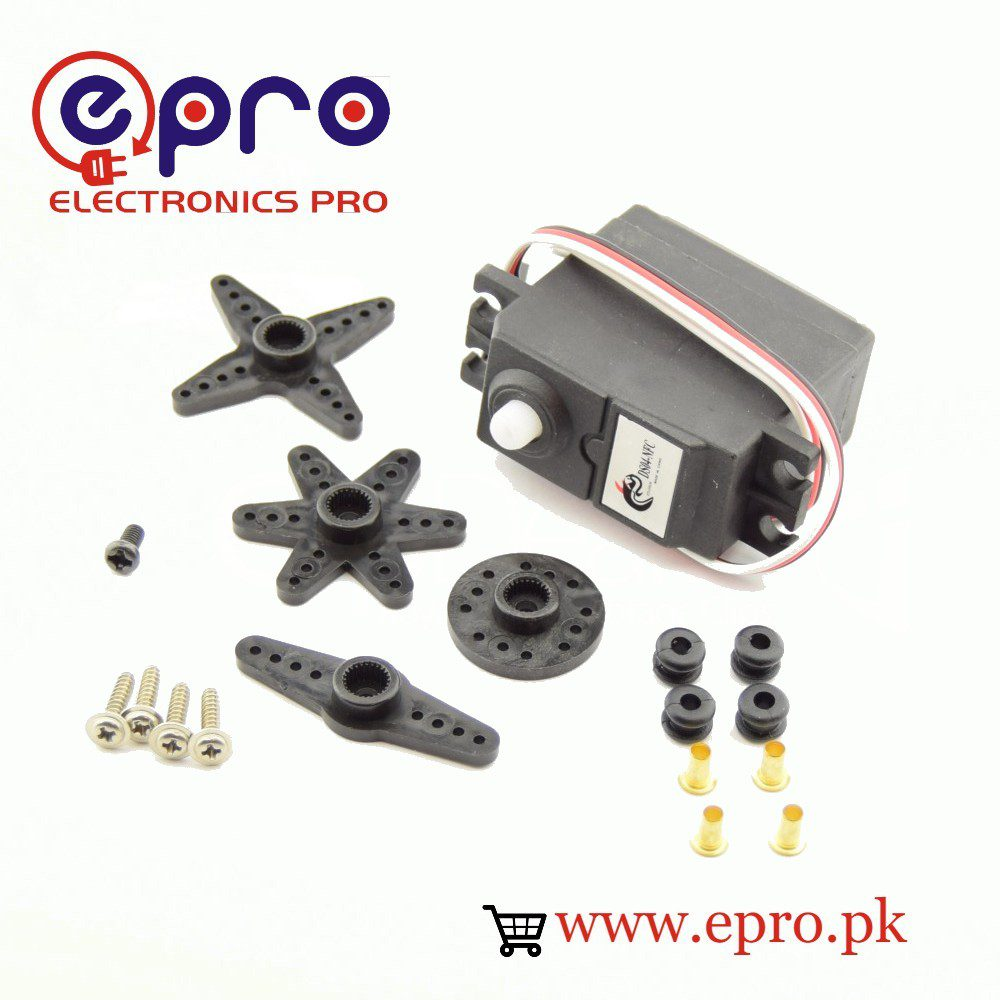450.00

0 out of 5
(0)
SKU: N/A

## Specifications:

Its model is DS04-NFC.

It is for aerospace model or cars

It is also for robots.

Its weight is 38g.

Its dimensions are 40.8 x 20 x 39.5m

Its torque is 5.5kg / cm when we apply 4.8V.

Its speed is 0.22 sec / 60 when we apply 4.8V.

Its operating voltages are 4.8V to 6V.

Its operating temperature is 0 degrees to 60 degrees.

Its current is less than 1000mA.0 out of 5
(0)

## Specifications:

Its model is DS04-NFC.

It is for aerospace model or cars

It is also for robots.

Its weight is 38g.

Its dimensions are 40.8 x 20 x 39.5m

Its torque is 5.5kg / cm when we apply 4.8V.

Its speed is 0.22 sec / 60 when we apply 4.8V.

Its operating voltages are 4.8V to 6V.

Its operating temperature is 0 degrees to 60 degrees.

Its current is less than 1000mA.

SKU: N/A
450.000 out of 5
(0)

## Specifications:

Its model is DS04-NFC.

It is for aerospace model or cars

It is also for robots.

Its weight is 38g.

Its dimensions are 40.8 x 20 x 39.5m

Its torque is 5.5kg / cm when we apply 4.8V.

Its speed is 0.22 sec / 60 when we apply 4.8V.

Its operating voltages are 4.8V to 6V.

Its operating temperature is 0 degrees to 60 degrees.

Its current is less than 1000mA.

450.000 out of 5
(0)

## Specifications:

Its model is DS04-NFC.

It is for aerospace model or cars

It is also for robots.

Its weight is 38g.

Its dimensions are 40.8 x 20 x 39.5m

Its torque is 5.5kg / cm when we apply 4.8V.

Its speed is 0.22 sec / 60 when we apply 4.8V.

Its operating voltages are 4.8V to 6V.

Its operating temperature is 0 degrees to 60 degrees.

Its current is less than 1000mA.

450.00